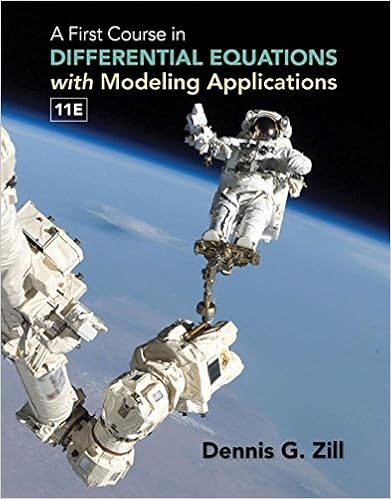# The general term in the sequence of partial sums is s

• Assignment
• 202
• 100% (3) 3 out of 3 people found this document helpful

This preview shows page 176 - 179 out of 202 pages.

##### We have textbook solutions for you!
The document you are viewing contains questions related to this textbook.The document you are viewing contains questions related to this textbook.
Chapter 6 / Exercise 3
A First Course in Differential Equations with Modeling Applications
ZillExpert Verified
The general term in the sequence of partial sums isSN=113+1214+1315+1416+ · · · +1N11N+1+1N1N+2=1+121N+11N+2=321N+1+1N+2.Finally,S=limN→∞SN=limN→∞321N+1+1N+2=32.37.EvaluateS=n=31n(n+3).solutionNote that1n(n+3)=131n1n+3so thatNn=31n(n+3)=13Nn=31n1n+3=131316+1417+15181619+ · · · +1N11N+2+1N1N+3=1313+14+151N+11N+21N+3Thusn=31n(n+3)=13limN→∞Nn=31n1n+3=1313+14+151N+11N+21N+3=1313+14+15=4718038.Find the total area of the infinitely many circles on the interval[0,1]in Figure 1.181412x10FIGURE 1solutionThe diameter of the largest circle is12, and the diameter of each smaller circle is12the diameterof the previous circle; thus, the diameter of thenth circle (forn1) is12nand the area isπ12n+12=π4n+1.
##### We have textbook solutions for you!
The document you are viewing contains questions related to this textbook.The document you are viewing contains questions related to this textbook.
Chapter 6 / Exercise 3
A First Course in Differential Equations with Modeling Applications
ZillExpert Verified
Chapter Review Exercises1475The total area of the circles isn=1π4n+1=π4n=114n=π4·14114=π12.In Exercises 39–42, use the Integral Test to determine whether the infinite series converges.39.n=1n2n3+1solutionLetf (x)=x2x3+1. This function is continuous and positive forx1. Becausef (x)=(x3+1)(2x)x2(3x2)(x3+1)2=x(2x3)(x3+1)2,we see thatf (x) <0 andfis decreasing on the intervalx2. Therefore, the Integral Test applies on theintervalx2. Now,2x2x3+1dx=limR→∞R2x2x3+1dx=13limR→∞ln(R3+1)ln 9= ∞.The integral diverges; hence, the seriesn=2n2n3+1diverges, as does the seriesn=1n2n3+1.40.n=1n2(n3+1)1.01solutionLetf (x)=x2(x3+1)1.01. This function is continuous and positive forx1. Becausef (x)=(x3+1)1.01(2x)x2·1.01(x3+1)0.01(3x2)(x3+1)2.02=x(x3+1)0.01(21.03x3)(x3+1)2.02,we see thatf (x) <0 andfis decreasing on the intervalx2. Therefore, the Integral Test applies on theintervalx2. Now,2x2(x3+1)1.01dx=limR→∞R2x2(x3+1)1.01dx= −10.03limR→∞1(R3+1)0.01190.01=10.03·90.01.The integral converges; hence, the seriesn=2n2(n3+1)1.01converges, as does the seriesn=1n2(n3+1)1.01.41.n=11(n+2)(ln(n+2))3solutionLetf (x)=1(x+2)ln3(x+2). Using the substitutionu=ln(x+2), so thatdu=1x+2dx, we have0f (x) dx=ln 21u3du=limR→∞ln 21u3du=limR→∞12u2Rln 2=limR→∞12(ln 2)212(lnR)2=12(ln 2)2Since the integral off (x)converges, so does the series.42.n=1n3en4solutionLetf (x)=x3ex4. This function is continuous and positive forx1. Becausef (x)=x34x3ex4+3x2ex4=x2ex434x4,
1476C H A P T E R10INFINITE SERIES(LT CHAPTER 11)we see thatf (x) <0 andfis decreasing on the intervalx1. Therefore, the Integral Test applies on theintervalx1. Now,1x3ex4dx=limR→∞R1x3ex4dx= −14limR→∞eR4e1=14
•••